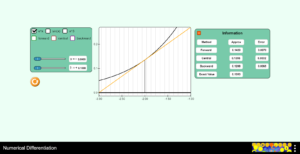# Chapter 02 Numerical Differentiation Divided Differences Simulation

Description:  This simulation depicts the central, backward, and forward divided-difference methods for estimating the first derivative of a set of simple functions.  The student can explore the true errors for each of the methods and see the graphical interpretation of the methods as compared to the exact solution.Keywords:  differentiation, data, slope, differential calculus, numerical differentiation

Learning Objectives:  After successful completion of running the simulation with proper questions asked by the instructor, the student would be able to 1) infer the effect of step size on the accuracy of central, backward, and forward divided difference methods of estimating the first derivative of a function, 2) infer how effect size affects the accuracy of backward and forward difference methods differently than central divided difference methods of estimating the first derivative of a function.

Full Resources:  The full resources for the topic of numerical differentiation are given here which include textbook content, a PowerPoint presentation, a multiple-choice test, audiovisual lectures, and application examples.